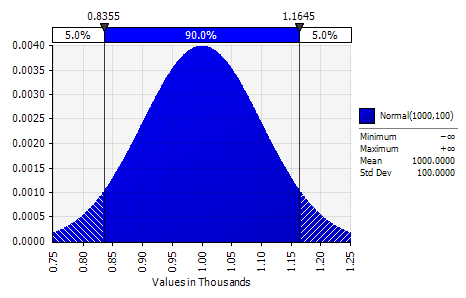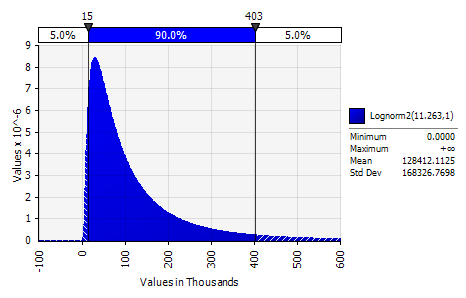# The Flaw of Averages

•Pitfalls of Averages in Risk Management and Claims Applications

We all know the big three: counts, total incurred and average.  The first two come directly from your claim data and the average is simply computed by dividing the total incurred by the claim count.  They are valuable statistics and provide useful insights—especially when you compare results over time and among your divisions.   So far, so good, but what does this “average” really tell us?

The first pitfall is that when we hear the word “average,” our immediate reaction is to think of a normal distribution.  Now normal distributions (shown here) are incredibility useful and convenient.  Why?  Because the expected value, median (where half the observations are greater and half are smaller), and the mode (point of highest probability) are equal to the average.  Great!  But, and it is a big But, normal distributions are rare indeed in risk management applications including severity.What we more commonly confront are distributions that look more like:We don’t need to quibble about all the details, but remember two things:  first, the substantial preponderance of claims is concentrated in the lower values, and second, the average (as computed above) is profoundly influenced by the relatively small number of large-valued claims.  Rather than bury you in statistics, here is an exercise for you to try.

Take your currently-valued WC claims for 2010:  first compute their average, and then sort the claims from largest to smallest.  Count how many claims have a value below the average and compute the ratio of that number to the total count.  I will be surprised if that value is less than 70% to 80%.  In other words, a high fraction of claims is less than the average.  Next count the top 5% of claims and compute the percentage of incurred associated.